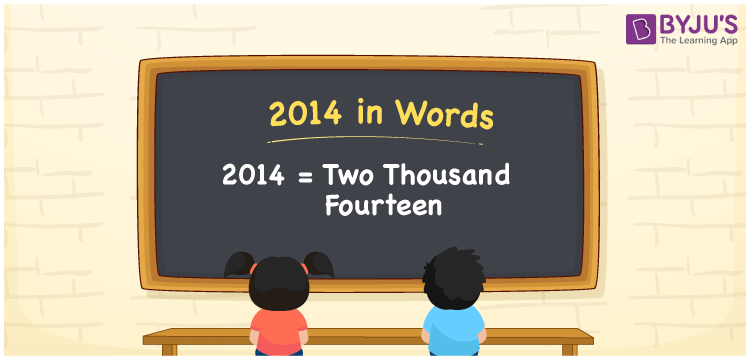# 2014 in Words

Two Thousand Fourteen” is represented by the number 2014. As we know, 2014 is a cardinal number, since it is used to count things and express values. The place value system can be used to convert the number 2014 to words. If you purchased clothes worth Rs. 2014, You can write it as “I purchased clothes worth Rs. Two thousand fourteen”. Let us take a look at how 2014 is spelt in words, as well as the method used in this article.

 2014 in Words: Two Thousand Fourteen. Two Thousand Fourteen in Numerical Form: 2014.

## 2014 in English Words## How to Write 2014 in Words?

The place value system can be used to convert the number 2014 to words. The table below shows the place values for the number 2014.

 Thousands Hundreds Tens Ones 2 0 1 4

The expanded form of 2014 is as follows:

= 2 × Thousand + 0 × Hundred + 1 × Ten + 4 × One

= 2 × 1000 + 1 × 10 + 4 × 1.

= 2000 + 10 + 4

= 2014

= Two thousand fourteen

Hence, 2014 in words is two thousand fourteen.

2014 in words – Two thousand fourteen

Is 2014 an odd number? – No

Is 2014 an even number? – Yes

Is 2014 a perfect square number? – No

Is 2014 a perfect cube number? – No

Is 2014 a prime number? – No

Is 2014 a composite number? – Yes

## Frequently Asked Questions on 2014 in Words

Q1

### Write 2014 in words.

2014 in words is two thousand fourteen.

Q2

### Simplify 2000 + 14, and express in words.

Simplifying 2000 + 14, we get 2014. Hence, 2014 in words is two thousand fourteen.

Q3

### How to write two thousand fourteen in numbers?

Two thousand fourteen in numbers is 2014.